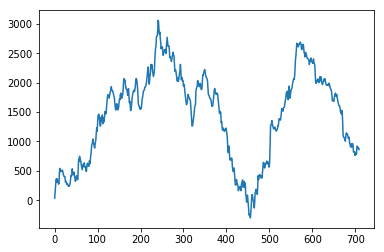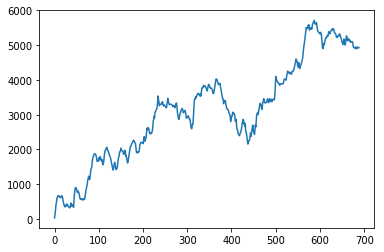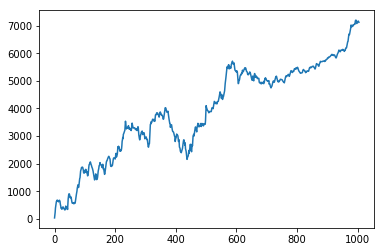### Trading Decisions of Your Stone Age Grandpa can Make You Money in FOREX

Why Ferrari or Rolex does not price their products at 149.999 or 12.999 but most of the items you see in your supermarket is priced like 4.99? Because they never like to be positioned as a bargain. Did you know that we tend to chose the price with less syllables even if the two prices have the same written lenght? These are some of the pricing strategies used by marketers.

This is a very interesting topic and you can even find yourself deep in neuroscience while reading about it. Check this site, it gives 42 pricing methods to influence your brain, crazy, https://www.nickkolenda.com/psychological-pricing-strategies/

We also deal with prices when trading and I believe there are some sub-concious forces in play. Knowing some articles on things like the effect of round numbers in trading, I see some more potential here and I find this worth digging more not as standalone trading strategies but more as filters. At the end of the day, no one knows how the price feed effects the primitive you sitting inside you.

Among the ones I have tested out of this 42 methods outlined at the above link, I want to share with one that can be applied as a filter.

In :
#do the imports
import pandas as pd
import numpy as np
import matplotlib.pyplot as plt
import bulk_tester as bt
%matplotlib inline
import datetime


Get price data for the Back Test period(BT) which is between 01.01.2009 and 01.01.2014 (M15 bars)

In :
dftest = bt.get_data('EURUSD', 'BT', 'original')


Now, I want to short EURUSD for this period and I want to do this when the market is calm, lets say I believe that signal to noise ratio is better when the market is calm. I want to short when the spread is smaller than 1.1 and volume is smaller than 300. Here 300 is the volume provided by OANDA and it shows how many ticks make up a bar, an indication of fragmented activity.

Before coding the trading logic, I want to get a list of bars and use it as a control group before I apply any trading logic. I get the indexes of every 10th bar. For the backtesting period, we have 12807 such bars in hand.

In :
index_of_entry = []
b = 0#this is the loop counter

#loop the frame
for index, row in dftest.iterrows():
#this is mostly randomly selected entry points at every 10 bar, the control group
if b % 10 == 0:
index_of_entry.append(b)

b += 1

In :
len(index_of_entry)

Out:
12807

Then I run a quick and dirty backtest to see the performance of this selected entry points. The trading rule here is this:

• short at the index if spread < 1.1 and volume < 300 (these are optimized by hand for the BT period)
• close after 50 bars (optimized by hand for BT period)
In :
a = index_of_entry
pnl = []
lag = 50#periods to close the position

for b in range(0,len(a)):

if a[b] + lag < len(dftest) and 10000*(dftest.Ask[a[b]] - dftest.Bid[a[b]]) < 1.1 and dftest.volume[a[b]] < 300:

pnl.append(pips)

print("Profit in Pips: ", np.sum(pnl))
plt.plot(np.cumsum(pnl))

Profit in Pips:  861.9

Out:
[<matplotlib.lines.Line2D at 0x11a34f198>]Not good right. Now I apply my stoneage grandpa filter and re select the entry points. Here Bid price has a 5 at the end.

In :
index_of_entry = []
b = 0#this is the loop counter

#loop the frame
for index, row in dftest.iterrows():
#get bid
bid = row["Bid"]
#this is if there is number 5 at  of the bid
if len(str(bid)) > 6 and int((str(bid))) in (5,5):
index_of_entry.append(b)

b += 1

In :
len(index_of_entry)

Out:
12681

And apply the same trading logic. What an improvement.

In :
a = index_of_entry
pnl = []
lag = 50#periods to close the position

for b in range(0,len(a)):

if a[b] + lag < len(dftest) and 10000*(dftest.Ask[a[b]] - dftest.Bid[a[b]]) < 1.1 and dftest.volume[a[b]] < 300:

pnl.append(pips)

print("Profit in Pips: ", np.sum(pnl))
plt.plot(np.cumsum(pnl))

Profit in Pips:  4920.6

Out:
[<matplotlib.lines.Line2D at 0x11edfb160>]And lets see what we have for all data covering unseen out of sample data(01.01.2009 to 05.05.2017) Here I simly copy pasted the same code above.

In :
#get price data for the entire set: between 01.01.2009 and 05.05.2017, M15 bars
dftest = bt.get_data('EURUSD', 'ALL', 'original')

In :
index_of_entry = []
b = 0#this is the loop counter

#loop the frame
for index, row in dftest.iterrows():
#get bid
bid = row["Bid"]
#this is if there is number 5 at  of the bid
if len(str(bid)) > 6 and int((str(bid))) in (5,5):
index_of_entry.append(b)

b += 1

In :
a = index_of_entry
pnl = []
lag = 50#periods to close the position

for b in range(0,len(a)):

if a[b] + lag < len(dftest) and 10000*(dftest.Ask[a[b]] - dftest.Bid[a[b]]) < 1.1 and dftest.volume[a[b]] < 300:

pnl.append(pips)

print("Profit in Pips: ", np.sum(pnl))
plt.plot(np.cumsum(pnl))

Profit in Pips:  7117.9

Out:
[<matplotlib.lines.Line2D at 0x11e60e278>]I have to make a note here. When you backtest this properly, you will not see an equity curve like this, it will be still up but with flat periods of no activity. The reason is that we have are looking at the cumulative pips return graph with number of trades in the x axis. In an equity curve, you see how your capital changes in equally spaced time.

If you find this post usefull, please like this on Quantocracy!

1.Hey, if in period = x, the algo shorts, but in period = x+10, and the trading logic conditions still hold, the algo will short yet again?

1.Hi Vinitrinh,

Thank you for the nice words.

You are correct, this algo holds multiple positions at time t. But to be honest, I did not engineer it for optimum performance that much, my point here is the effect of a single digit when used as a filter.

If you test it with a single position at time t logic, let me know the outcome.

Thanks,

QTJ

2.Very good post, I've been an FX and Equities trader for a while and it completely makes sense from the trader's perspective: we tend to round up levels all the time to simplify our strategies.

1.Thank you Esteban. I am using this one as a filter in my trading strategies and it works. Also, i encourage you to look at the other biases mentioned at the link in the post.

3.Good one! I found this information very useful. To make profit it is important to understand market and all aspects of trading.

4.Really got a useful blog to read today,keep posting more like this.